# Master basics of machine learning by solving a hackathon problem

## Learn how to solve regression problem step by step and gain decent rank in hackathon leaderboardsHackathons are a good way to learn and implement new concepts in a short span of time. Today we are going to cover basic steps in machine learning and how to get a good accuracy for a regression problem while trying to achieve a decent rank using a dataset from MachineHack Hackathon.

The basic steps in any solving any machine learning problem are:-

1. Identifying the target and independent features
2. Cleaning the data set
3. Feature Engineering
4. Feature Encoding and Scaling
5. Feature selection
6. Check distribution of target variable
7. Get insights from graphs for fine-tuning features
8. Model application and hyper-parameter tuning
9. Combining different models

Phew…That’s a lot of steps to cover and beginners can get easily intimidated…so let’s deep dive into the problem and cover one step at a time…

You can download the dataset by creating an account on MachineHack website and download the dataset by registering for the following hackathon:- Flight ticket price prediction

Now there are 3 files which are provided in the zip file you get from the hackathon:-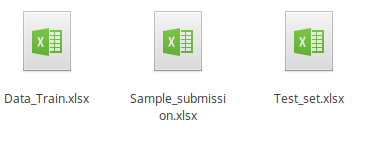Files provided in the dataset: 1)Data_train.xlsx 2)Sample_submission 3)Test_set

Here `Data_Train.xlsx` contains the data-set using which we need to train the model, `Sample_submission` as the name suggests specifies the format in which output needs to be submitted in the hackathon and `Test_set` is the data-set on which need we need to apply our model in order to predict flight ticket prices on the basis of which our score in the hackathon will be evaluated.

Now one thing we need to keep in mind is whichever transformations we are going to apply on `Data_Train` data-set features the same would need to be applied on `Test_set` data-set so that the model gets a similar type of inputs from both of them.

Next, download the juptyer notebook from this GitHub repository which covers all the above steps in detail:

HackathonProblemSolution

Well if you have come up to this step that means you are serious about learning new things so start some nice music in another tab to get into the zone and let’s begin…

1. Identifying target and independent features

First step in solving any machine learning problem is to identify the source variables (independent variables) and the target variable (dependent variable).

Target variable, in a machine learning context, is the variable should be the output. For example, it could be binary 0 or 1 if you are classifying or it could be a continuous variable if you are doing a regression.

Independent variables (also referred to as Features) are the input for a process that is being analyzed.

We have been provided the following information about the dataset on the official website:-

Size of training set: 10683 records

Size of test set: 2671 records

FEATURES:
Airline: The name of the airline.

Date_of_Journey: The date of the journey

Source: The source from which the service begins.

Destination: The destination where the service ends.

Route: The route that was taken by the flight to reach the destination.

Dep_Time: The time when the journey starts from the source.

Arrival_Time: Time of arrival at the destination.

Duration: Total duration of the flight.

Total_Stops: Total stops between the source and destination.

Price: The price of the ticket

Let us import the dataset in the jupyter notebook using `pd.read_excel` command. You can find information about importing various kind of files in pandas here.

Let us use `df.head()` command in pandas to get an idea about the columns in our dataset. Just keep in mind that I have kept the name of the dataset on which we are training the model as `df_train`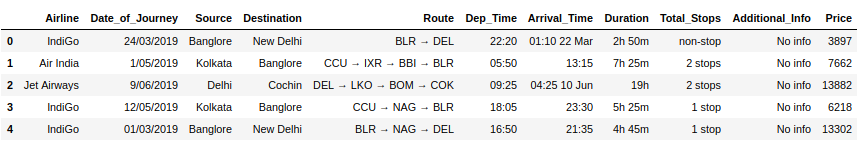df.head() is used to print first 5 rows of the dataset

Here we can see that price column is the target variable and since it has continuous values i.e. which cannot be classified into specific categories, the problem is a supervised regression one.

2. Cleaning the dataset:

First, let us check the number of missing values in our dataset using `df.isnull()` command:-

Check null values

Since we have only one null value in our dataset I am simply removing it, as making efforts to impute a single value does not seem like a good option.

But keep in mind that the general rule of thumb is if more than 30% values in any particular column are missing then we can exclude that column.

Removing null values is easy in pandas by running the below command:-

Here `inplace` parameter is used to do the operation implicitly i.e. apply the operation directly to the specified dataframe.

Next, we should check if our dataset has any duplicates rows and drop them:-

The `df.duplicated()` is used to find the total duplicate entries in our dataframe.

Check duplicate rows

Next, we need to drop the duplicate entries by running the following command:-

```#remove duplicate rows in training datasetdf_train.drop_duplicates(keep='first',inplace=True)
```

In the `drop_duplicates` command above, `keep='first'` option allows us to keep the first occurrence of the row values while removing all the subsequent occurrences.

You can now also remove unnecessary columns simply by taking a look at dataset at this step only. In the present dataset, there were no such columns so we can proceed ahead.

If you observe the steps taken in the Data cleaning section of the notebook we have merged repeating values in `Additional_Info` column and renamed values in Total_Stops column.

3) Feature Engineering:-

Feature engineering is the process of using domain knowledge of the problem and a bit of common sense to create new features which can increase the predictive power of machine learning models. This step does require quite a bit of imagination, critical thinking about the input features and using a part of your creative side.

The `Date_of_Journey` column is in itself not quite useful but we can create new features from it like whether the flight was on a weekend or not, the day of the week and month.

Feature engineering

Whoa…That looks like a complicated piece of code to understand…Trust me I did not come up with it by myself and can probably never will. But this is where StackOverflow came to the rescue. You can read further about the code from the below links since this will help you in

a)Understanding various ways in which same task can be achieved in python

b)Just in case tomorrow this code snippet becomes redundant or doesn’t work for you guess which site is going to help you in finding the solution 😉

2) Check if a day falls on a weekend in pandas

3) Get year, month and day from a datetime variable in pandas

We then convert the duration column values into minutes:-

Feature engineering on duration column

If you take a look at `Duration` column the format of values is like `2h 50m` with some rows containing only hour values while some only minutes(Like how could someone enjoy a flight which ends in less than an hour but that’s a discussion for some other day)

So in the above code, the initial for loops separate the hours and minutes parts while the second for loop is used to convert the duration into minutes.

P.S. I even tried converting into seconds for improving the accuracy but please don’t follow such stupid steps unless you have loads of free time and hell-bent on improving rank on the leader-board 👻

Next, we have created a new column called called `Duration_minutes` and dropped the original `Duration` column from the dataframe.

I have also performed similar steps on Arrival_Time column which you can follow up in the notebook.

4) Feature Encoding and Scaling:-

We usually perform feature scaling and encoding on independent i.e input variables so let us separate the target and input variables from each other in the dataframe.

Split the dataset

Here `X` contains the data frame of input features while `y` is our target variable. I will cover the reason for applying log transformation to `y` in the next section. For now, let us focus on our input variables dataframe viz `X`

The first step is to split input variables into categorical and numerical variables.

Categorical variables contain a finite number of categories or distinct groups. Categorical data might not have a logical order. For example, categorical predictors include gender, material type, and payment method.

Numerical variables as the name suggest contains continuous or discrete numerical values.

We can make the split using `df.select_dtypes()` method:-

select_dtypes is used to separate numerical and categorical features

In the case of categorical features, we can either apply label encoding or one hot encoding.

Implementation

When to use label encoding vs Label encoder

In the current dataset, we have done label encoding for categorical features.

Label encoding variables

In the case of numerical features, we can perform different types of scaling like MinMax, StandardScaler or BoxCox tranformation.

I tried variations of standard scaling, min-max scaling and boxcox transformation for numerical ones. In the end, boxcox transformation gave the best accuracy score.

Boxcox transformation

The Box-Cox transformation is a family of power transform functions that are used to stabilize variance and make a dataset look more like a normal distribution. Explanation about box-cox transformation can be found here.

The `lam` variable specifies the type of transformation to be applied:-

• lambda = -1. is a reciprocal transform.
• lambda = -0.5 is a reciprocal square root transform.
• lambda = 0.0 is a log transform.
• lambda = 0.5 is a square root transform.
• lambda = 1.0 is no transform.

Once we are done with above transformations we join the categorical and numerical features back to get a set of transformed input variables.

5) Feature selection:-

We can apply tree-based regression models like random forest regressor, extra trees, and xgboost to get feature importances.

For example, RandomForestRegressor model can be applied on the dataset as follows:-

Random Forest

We first split the dataset into training and testing samples using `train_test_split` method. The `test_size` param specifies the proportion of training and test data. A value of 0.3 i.e 30% splits the data into a 70:30 ratio of training and test data. We then define RandomForestRegressor model and apply it on the training sample(X_train, y_train).

Then we make prediction on testing input sample(X_test) and compare it with the original target sample(y_test) to get various accuracy metrics. I will cover the accuracy metrics in further sections of the article.

For now let us see feature importance predicted by the model using the function below:-

Feature Importances

In the above function, we create a new dataframe from feature importances provided by the model in descending order of importance.

Then we create a horizontal bar plot using matplotlib library to see the feature importances visually.

In the case of random forest regressor, the output of the function is:-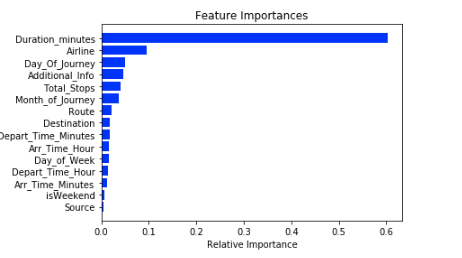Feature Importances

As we can see the duration column has the highest importance while source city from which flight originated has the lowest feature importance.

We can either select features manually from the graphs generated above or we can use `SelectFromModel` module from `sklearn` to select the most appropriate features automatically.

I tried running the model on selected features according to above feature importances but accuracy reduced slightly which might be great if we were deploying the model in real-world scenario since the model is now a bit more robust but for a hackathon, accuracy matters the most so I ended up keeping all the features in the end.

7) Check distribution of target variable:-

We should check the distribution of the target variable in a regression problem using distribution plot. If it is skewed then application of log, exponent or sqrt transform can help in reducing the skewness to get a normal distribution.

The distribution of the target variable in our case initially was a bit right skewed:-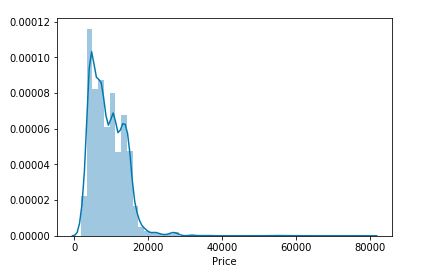After applying log transformation, it was normally distributed:-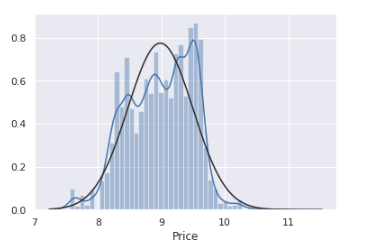Normalized distribution

The log transformation did improve the overall accuracy of the model at the end which was the reason I applied log transformation to target input at the beginning of Step 4 above.

8) Get insights from graphs:-

Check variation of target column with respect to input variables and distribution of various input variables:-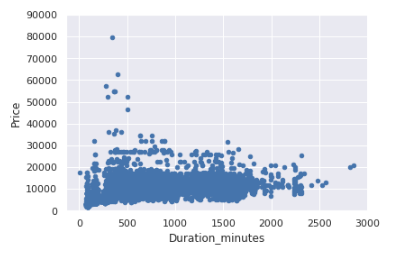Scatter plot

The price should have increased with increase in duration but this was not the case here.

Next, we check the variation of price against the total number of stops:-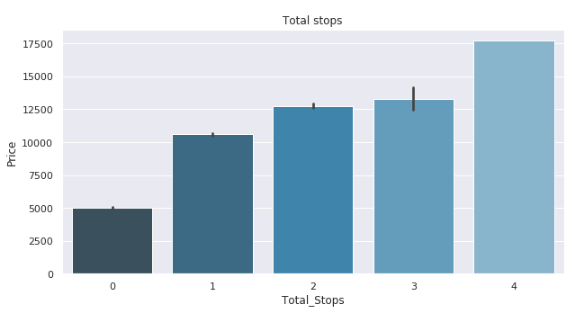Total stops vs Price

Insight from the above graph:-
As expected, the price of flight tickets is higher for flights with a greater number of stops

The distribution of numerical features can be checked using a histogram plot while the distribution on categorical features can be checked using a bar plot or box plot.

In case of categorical features check if any of the column values can be combined together while in case of numerical features check if distribution can be normalized i.e. evenly distributed.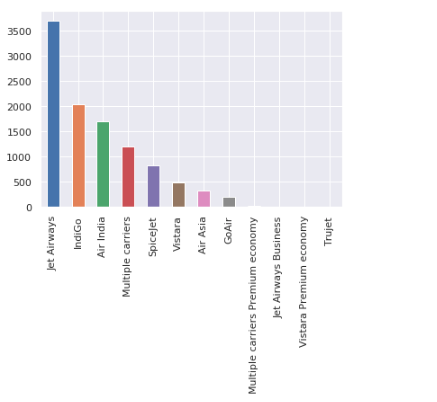Airlines frequency bar plot

I went back into categorical features and clubbed together last four airline categories later.

Similar steps were followed for `Additional_Info` column:-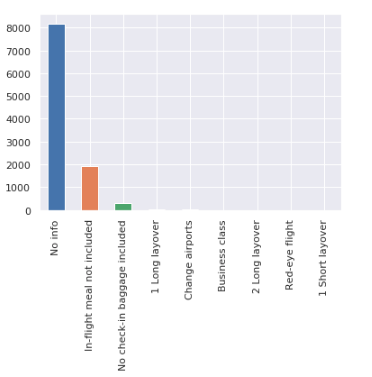You can find histograms of numerical features in the jupyter notebook and make necessary transformations if required.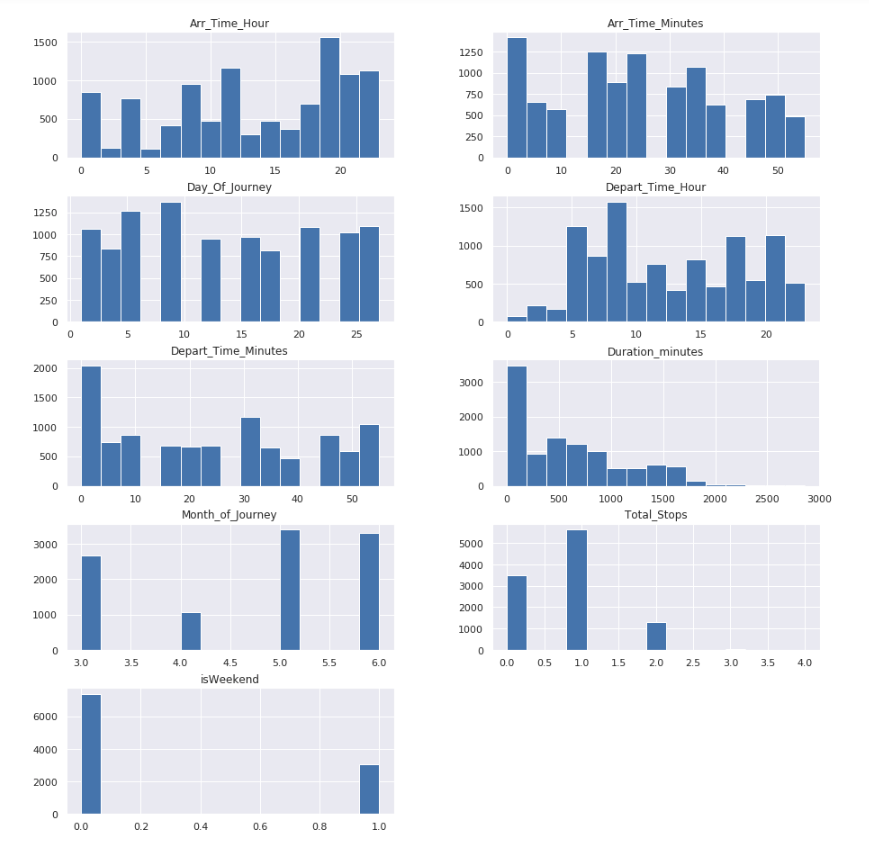9)Model application and hyper-parameter tuning:-

After trying different kinds of regression models I found ExtraTrees, RandomForest, and XGboost gave slightly better accuracy than other models so it was time to perform hyperparameter tuning on all 3 of them to improve accuracy further.

In machine learning, a hyperparameter is a parameter whose value is set before the learning process begins. We need to come up with optimum values of hyperparameters because these values are external to the model and their value cannot be estimated from data.

Hyperparameter tuning is the process of choosing a set of optimal hyperparameters for a learning algorithm. The two common approaches to do hyperparameter tuning are GridSearchCV and RandomisedSearchCV.

Although GridSearchCV is exhaustive, RandomisedSearchCV is helpful to get a range of relevant values quickly. More information about them can be found here.

Randomized Search CV

In the case of the RandomForest algorithm, the following article from towards data science helps us to understand how to do hyperparameter tuning properly:- Article Link

A similar approach can be applied for ExtraTrees and XGBoost Regressor Models.

10) Combining different models

Stacking is an ensemble learning technique in which we can combine multiple regression models via a meta-classifier or a meta-regressor. The base level models are trained based on a complete training set, then the meta-model is trained on the outputs of the base level models as input features.

In the last step I combined above three models using the stacking technique to improve overall accuracy:-

Stacked model

Here base models used are ExtraTrees, Random Forest and XGBoost Regressor while the meta-model used is Lasso. You can learn more about how to implement stacking here.

Next, we fit the stacked model and make predictions on the test data sample

Fit the model

Next, I have created a simple function to print all available accuracy metrics in the regression model:-

Print accuracy report

Once we have our stacked model trained we simply need to apply the model on prediction dataset and submit the values to the hackathon website, which finishes of all the essential steps required in solving a machine learning problem.

The final metrics of the stacked model are:-

Rsquared measures the strength of the relationship between your model and the dependent variables on a 0–1 scale. The information about other metrics can be found here.

Hope you got at least a bit of a grasp on how to approach a supervised machine learning problem after finishing all the steps above. If you have any doubts, suggestions or corrections do mention them in the comments section and if you like this article show a bit of appreciation by sharing it with others.

And last but not the least happy coding 🤓

Categories Featured Excerpt